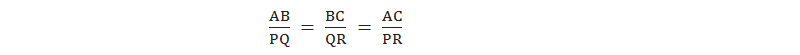# Similar Triangles## Similar Triangles

Two triangles are said to be similar if they are exactly same by their shape. Two triangles of the same shape is mean three angles of one triangle are correspondingly equal to the three angles of the other triangle. The symbol for similarity is ~.

For example: In the given triangles ABC and PQR, A = P, B = Q and C = R. So the triangles ABC and PQR are similar. It is written as ΔABC ~ ΔPQR.

When two triangles are similar then their corresponding sides are in proportion i.e### Conditions for Similarity

#### Condition - 1. Angle-Angle-Angle (AAA) axiom

When three angles of any triangle are equal to the corresponding three angles of another triangle, then they will be similar by AAA axiom.
In the given triangles ABC and PQR, A = P (A), B = Q (A), C = R (A). So, ΔABC ~ ΔPQR by AAA axiom. Corresponding sides of similar triangles are proportional, i.e.

#### Condition - 2.

When three sides of a triangle are proportional to the corresponding three sides of another triangle, then they are similar.
In the given triangles ABC and PQR,

So, ΔABC ~ ΔPQR. Corresponding angles of similar triangles equal, i.e. A=P, B=Q and C=R.

### Workout Examples

Example 1: In ΔABC, XY//BC. Prove that:

Solution: Here,
In ΔAXY and ΔABC,
i.       AXY = ABC (A) -----------> corresponding angle
ii.     AYX = ACB (A) -----------> corresponding angles
iii.    XAY = BAC (A) ------------> common angle
ΔAXY ~ ΔABC ----------------> by AAA axiom
Proved.

Example 2: In the adjoining figure DE//BC. Find the values of x and y.

Solution: Here,
i.        DAE = BAC (A) -----------> common angle
ii.      DEA = ACB (A) -----------> corresponding angles
iii.    ADE = ABC (A) ------------> remaining angles
ΔADE ~ ΔABC ----------------> by AAA axiom
or,  3x = x + 18
or,  3x – x = 18
or,  2x = 18
or,  x = 18/2
or, x = 9
Again, taking second and third ratios,
or,  y = 18
x = 9cm and y = 18cm

You can comment your questions or problems regarding similar triangles here.